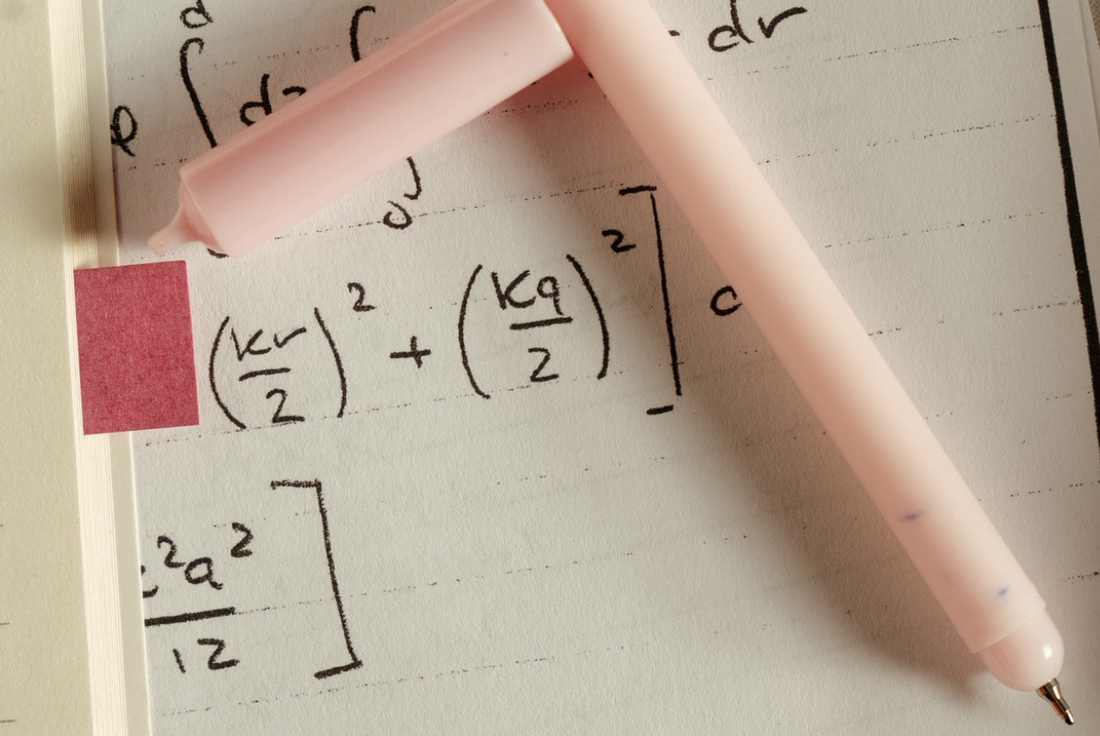# GMAT Math Tips## Quantitative Reasoning Section

The GMAT math portion of the exam includes 31 multiple-choice questions that test your mathematical reasoning, ability to interpret graphic data, and quantitative problem-solving skills.

You'll have 62 minutes to answer the GMAT's math questions, and you cannot use a calculator while completing this section of the exam.

## GMAT Quant Scores

Determining your results for Quantitative Reasoning on the GMAT includes three factors:

• Question difficulty level

## Difficulty of Questions & Scoring

Answering a higher number of questions correctly at a greater difficulty level increases your GMAT Quant score. Results for this section range from 6 to 51, and the average GMAT math score is 40.7.

Since the test adapts according to your performance, preparing for GMAT Quant questions improves your chances of getting better results.

## Understanding the GMAT Math Section

During the exam, you'll encounter two types of GMAT Quant questions – Problem Solving and Data Sufficiency. While each type presents its own unique challenges, both require a basic understanding of arithmetic, elementary algebra, and standard geometry.

Keep in mind that your logic and analytical skills are the focus of this section, not your actual math skills.

### Problem Solving

These GMAT math questions test your ability to use analytical reasoning and logic to assess and solve quantitative problems. To demonstrate your understanding of the various mathematical concepts, you'll need to solve each question and determine which of the five possible answers is correct.

### Data Sufficiency

Data Sufficiency problems include a question along with two statements.

Solving these GMAT math questions demonstrates your ability to:

• Analyze a quantitative problem
• Determine which data is relevant
• Assess whether the available data is enough to solve the problem

The questions on this portion of the exam require you to combine the data in each problem with your knowledge of basic math and real-world facts to select the best of five possible answers.

### Concepts Behind the Questions

The following list includes a few concepts you may encounter when answering GMAT math questions. Keep in mind that there are additional ideas and principles beyond what is listed here, so consider GradPrep's GMAT test prep resources to ensure you're ready on test day:

• Converting fractions, decimals, percentages, and ratios
• Calculating roots and exponents
• Manipulating algebraic expressions
• Applying descriptive statistics such as mean, median, mode, and standard deviation
• Performing permutations and combinations
• Understanding and calculating probability
• Manipulating inequalities
• Solving linear and quadratic equations
• Demonstrating knowledge of triangles, quadrilaterals, circles, lines, and angles
• Calculating interest, discounts, profits, and rates

## Preparation

### Brushing Up on Old Skills

When it comes to the computation and application of math concepts, many test-takers need a refresher on even the most fundamental principles. Since the GMAT Quant portion of the exam covers a mix of basic and advanced arithmetic, algebra, geometry, and real-world application, it's crucial to review those concepts.

Assemble a collection of GMAT math practice questions and add them to your study schedule. Then, organize the problems into different sections, including basic arithmetic, algebra, geometry, and real-world concepts. From there, study each section until you feel prepared for test day. Remember that knowing how each concept works is more important than your ability to perform calculations.

## Study Resources

Online GMAT Test Prep